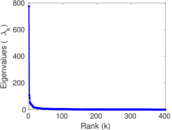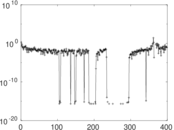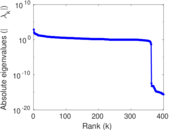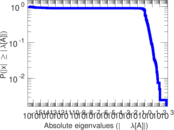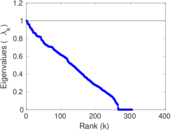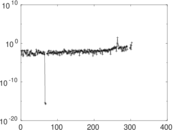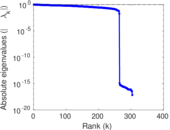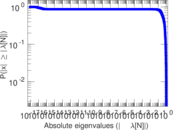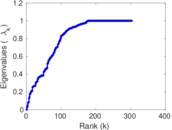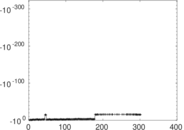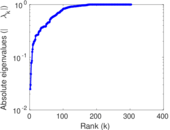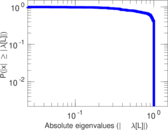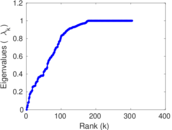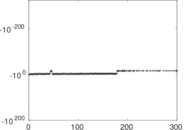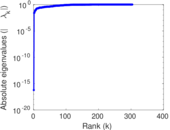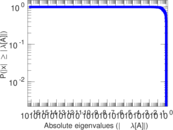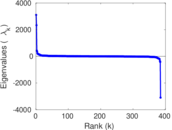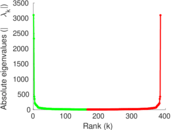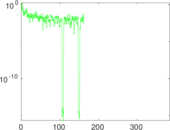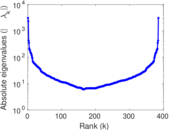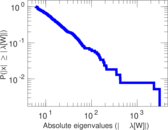# Wikiquote edits (da)

This is the bipartite edit network of the Danish Wikiquote. It contains users and pages from the Danish Wikiquote, connected by edit events. Each edge represents an edit. The dataset includes the timestamp of each edit.

 Code `qda` Internal name `edit-dawikiquote` Name Wikiquote edits (da) Data source http://dumps.wikimedia.org/ AvailabilityDataset is available for download Consistency checkDataset passed all tests Category Authorship network Dataset timestamp 2017-10-20 Node meaning User, article Edge meaning Edit Network formatBipartite, undirected Edge typeUnweighted, multiple edges Temporal dataEdges are annotated with timestamps

## Statistics

 Size n = 2,355 Left size n1 = 402 Right size n2 = 1,953 Volume m = 9,143 Unique edge count m̿ = 4,509 Wedge count s = 327,864 Claw count z = 29,417,586 Cross count x = 2,520,532,538 Square count q = 105,524 4-Tour count T4 = 2,168,834 Maximum degree dmax = 1,663 Maximum left degree d1max = 1,663 Maximum right degree d2max = 905 Average degree d = 7.764 76 Average left degree d1 = 22.743 8 Average right degree d2 = 4.681 52 Fill p = 0.005 743 17 Average edge multiplicity m̃ = 2.027 72 Size of LCC N = 2,036 Diameter δ = 13 50-Percentile effective diameter δ0.5 = 3.506 87 90-Percentile effective diameter δ0.9 = 5.320 35 Median distance δM = 4 Mean distance δm = 4.070 25 Gini coefficient G = 0.793 847 Balanced inequality ratio P = 0.173 411 Left balanced inequality ratio P1 = 0.123 482 Right balanced inequality ratio P2 = 0.231 653 Relative edge distribution entropy Her = 0.822 609 Power law exponent γ = 2.818 15 Tail power law exponent γt = 2.021 00 Tail power law exponent with p γ3 = 2.021 00 p-value p = 0.032 000 0 Left tail power law exponent with p γ3,1 = 1.711 00 Left p-value p1 = 0.556 000 Right tail power law exponent with p γ3,2 = 2.131 00 Right p-value p2 = 0.004 000 00 Degree assortativity ρ = −0.230 370 Degree assortativity p-value pρ = 2.200 87 × 10−55 Spectral norm α = 775.265 Algebraic connectivity a = 0.024 769 8 Spectral separation |λ1[A] / λ2[A]| = 7.178 94 Controllability C = 1,598 Relative controllability Cr = 0.688 200

## Plots

### Fruchterman–Reingold graph drawing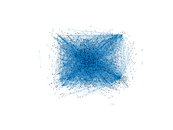### Degree distribution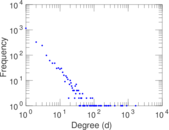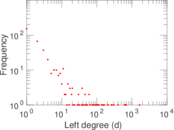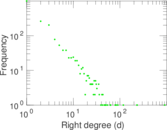### Cumulative degree distribution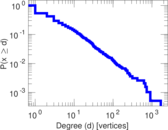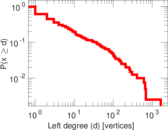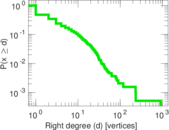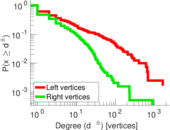### Lorenz curve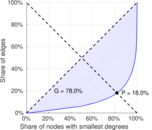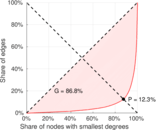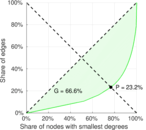### Spectral distribution of the adjacency matrix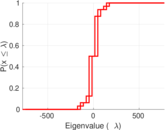### Spectral distribution of the normalized adjacency matrix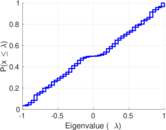### Spectral distribution of the Laplacian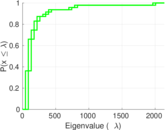### Spectral graph drawing based on the adjacency matrix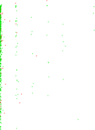### Spectral graph drawing based on the Laplacian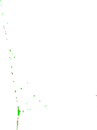### Spectral graph drawing based on the normalized adjacency matrix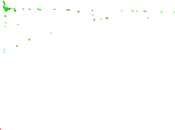### Degree assortativity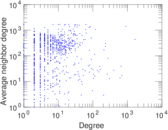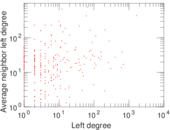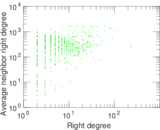### Zipf plot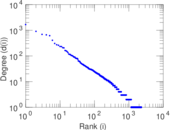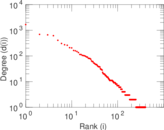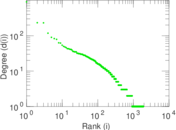### Hop distribution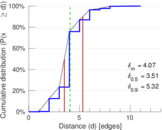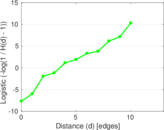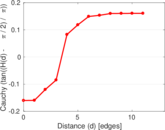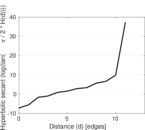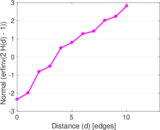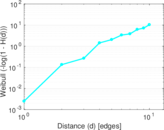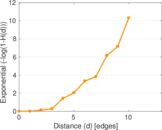### Double Laplacian graph drawing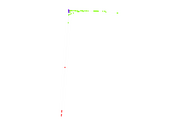### Delaunay graph drawing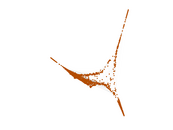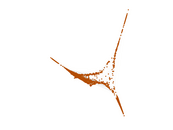### Edge weight/multiplicity distribution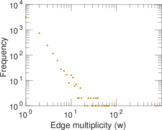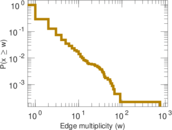### Temporal distribution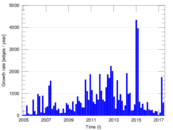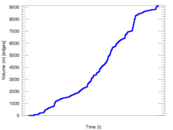### Temporal hop distribution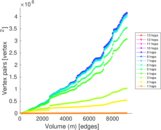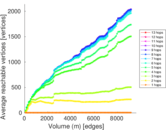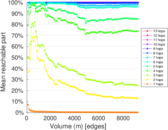### Diameter/density evolution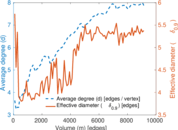### Matrix decompositions plots